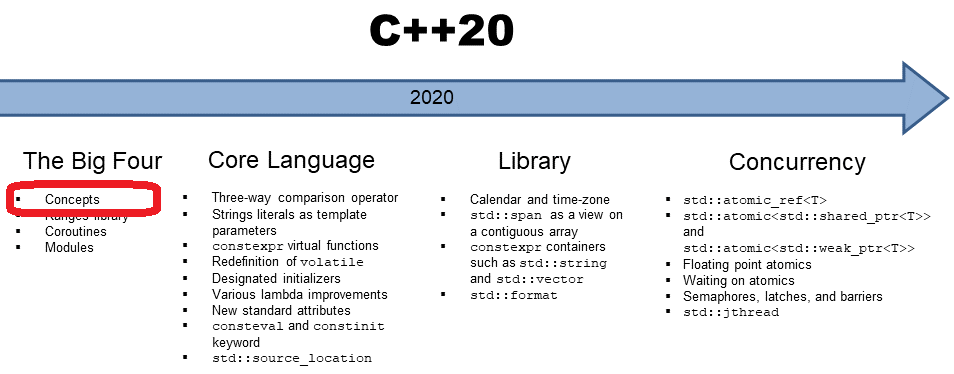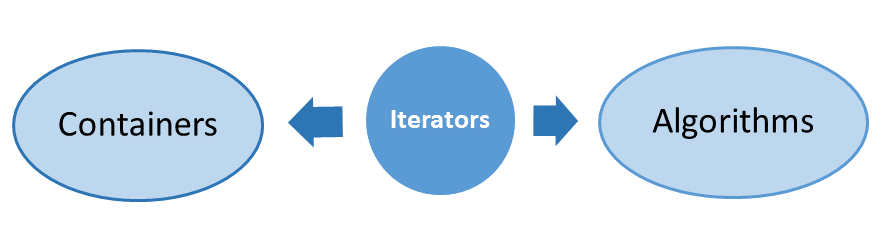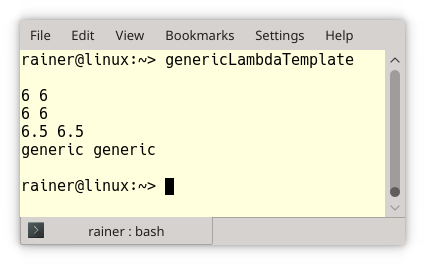# C++20: Concepts, the Placeholder Syntax

Today, I have a simple answer to a challenging question: Where can I use my concept? Concepts can be used where auto is usable.Before I write about the placeholder syntax and the new way to define function templates, I must make a detour. We have asymmetries in C++11/14.

## From Asymmetries in C++11/14 to Symmetries in C++20

I often have discussions in my C++ seminars that goes like this.

What is the Standard Template Library from the birds-eyes perspective? Generic containers can be manipulated with generic algorithms. The glue between these two disjunct components is iterators.##Modernes C++ Mentoring

Be part of my mentoring programs:

• "Fundamentals for C++ Professionals" (open)
• "Design Patterns and Architectural Patterns with C++" (open)
• "C++20: Get the Details" (reopens December 2023)
• Do you want to stay informed about my mentoring programs: Subscribe via E-Mail.

Generic containers and algorithms mean they are not bound to a specific type. Fine. Many of the algorithms can be parametrized by a callable. For example, std::sort has an overload that takes a binary predicate (callable). This binary predicate is applied to the elements of the container. A binary predicate is a function that takes two arguments and returns a boolean. You can use a function, a function object, or with C++11 lambda as a binary predicate.

### The Small Asymmetry in C++11

What’s wrong with the following program? (I know that we have the predefined predicate std::greater.)

```// lambdaCpp11.cpp

#include <algorithm>
#include <iostream>
#include <string>
#include <array>
#include <vector>

template <typename Cont, typename Pred>
void sortDescending(Cont& cont, Pred pred){
std::sort(cont.begin(), cont.end(), pred);
}

template <typename Cont>
void printMe(const Cont& cont){
for (auto c: cont) std::cout << c << " ";
std::cout << std::endl;
}

int main(){

std:: cout << std::endl;

std::array<int, 10> myArray{5, -10, 3, 2, 7, 8, 9, -4, 3, 4};
std::vector<double> myVector{5.1, -10.5, 3.1, 2.0, 7.2, 8.3};
std::vector<std::string> myVector2{"Only", "for", "testing", "purpose"};

sortDescending(myArray, [](int fir, int sec){ return fir > sec; });           // (1)
sortDescending(myVector, [](double fir, double sec){ return fir > sec; });    // (2)
sortDescending(myVector2, [](const std::string& fir, const std::string& sec){ // (3)
return fir > sec;
});

printMe(myArray);
printMe(myVector);
printMe(myVector2);

std::cout << std::endl;

}
```

The program has a std::array of int’s, a std::vector of double’s, and a std::vector of std::string‘s. All containers should be sorted in descending order and displayed. To ease my job, I create the two function templates sortDescending and printMe.Although containers and algorithms are generic, C++11 has only type-based lambdas. I have to implement the binary predicate for each data type (lines 1 – 3) and break, therefore, my generic approach.

With C++14, this asymmetry disappears. Containers and algorithms are generic, and lambdas can be generic.

### The Big Asymmetry in C++14

C++14 implements the previous program lambdaCpp11.cpp straightforward.

```// lambdaCpp14.cpp

#include <algorithm>
#include <iostream>
#include <string>
#include <array>
#include <vector>

template <typename Cont>
void sortDescending(Cont& cont){
std::sort(cont.begin(), cont.end(), [](auto fir, auto sec){   // (1)
return fir > sec;
});
}

template <typename Cont>
void printMe(const Cont& cont){
for (auto c: cont) std::cout << c << " ";
std::cout << std::endl;
}

int main(){

std:: cout << std::endl;

std::array<int, 10> myArray{5, -10, 3, 2, 7, 8, 9, -4, 3, 4};
std::vector<double> myVector{5.1, -10.5, 3.1, 2.0, 7.2, 8.3};
std::vector<std::string> myVector2{"Only", "for", "testing", "purpose"};

sortDescending(myArray);      // (2)
sortDescending(myVector);     // (2)
sortDescending(myVector2);    // (2)

printMe(myArray);
printMe(myVector);
printMe(myVector2);

std::cout << std::endl;

}
```

Fine? Yes and No. Yes, because I can use a generic lambda (line 1) for each data type in line 2. No, because I replaced one slight asymmetry in C++11 with a bigger asymmetry in C++14. The slight asymmetry in C++11 was that a lambda is type-bound. The big asymmetry in C++14 is that generic lambda introduced a new syntactic form to write generic functions, better known as function templates. Here is the proof:

```// genericLambdaTemplate.cpp

#include <iostream>
#include <string>

auto addLambda = [](auto fir, auto sec){ return fir + sec; }; // (1)

template <typename T, typename T2>                            // (2)
auto addTemplate(T fir, T2 sec){ return fir + sec; }

int main(){

std::cout << std::boolalpha << std::endl;

std::cout << addLambda(1, 5) << " " << addTemplate(1, 5) << std::endl;
std::cout << addLambda(true, 5) << " " << addTemplate(true, 5) << std::endl;
std::cout << addLambda(1, 5.5) << " " << addTemplate(1, 5.5) << std::endl;

const std::string fir{"ge"};
const std::string sec{"neric"};
std::cout << addLambda(fir, sec) << " " << addTemplate(fir, sec) << std::endl;

std::cout << std::endl;

}
```

The generic lambda in line 1 and the function template in line 2 produce the same results.This is the asymmetry in C++14. Generic lambdas introduce a new way to define function templates.

When I teach this in my seminars, I almost always get the question: Can we use auto in functions to get function templates? No with C++17, but yes with C++20.  In C++20, you can use constrained placeholders (concepts) or unconstrained placeholders (auto) in function declarations to get function templates. This means the asymmetry in C++ is gone with C++20.

### The Solution in C++20

Before I write about the new definition of function templates, I want to answer my original question: Where can I use my concept? Concepts can be used where auto is usable.

```// placeholdersDraft.cpp

#include <iostream>
#include <type_traits>
#include <vector>

template<typename T>                                   // (1)
concept Integral = std::is_integral<T>::value;

Integral auto getIntegral(int val){                    // (2)
return val;
}

int main(){

std::cout << std::boolalpha << std::endl;

std::vector<int> vec{1, 2, 3, 4, 5};
for (Integral auto i: vec) std::cout << i << " ";  // (3)

Integral auto b = true;                            // (4)
std::cout << b << std::endl;

Integral auto integ = getIntegral(10);             // (5)
std::cout << integ << std::endl;

auto integ1 = getIntegral(10);                     // (6)
std::cout << integ1 << std::endl;

std::cout << std::endl;

}
```

The concept Integral in line 1 can be used as a return type (line 2), in a range-based for-loop (line 3), or as a type for the variable b (line 4) or the variable integ (line 5). To see the symmetry, line 6 uses type-deduction with auto instead. I have to admit that no compiler is now available to compile the proposed concepts syntax I used in my example. The following output is, therefore, from GCC and the previous Concepts Technical Specification (Concepts TS).## What’s next?

My next post concerns the syntactic sugar we get with constrained placeholders (concepts) and unconstrained placeholders (auto) in C++20. I assume the function (template) getIntegral gives you a first impression.

Thanks a lot to my Patreon Supporters: Matt Braun, Roman Postanciuc, Tobias Zindl, G Prvulovic, Reinhold Dröge, Abernitzke, Frank Grimm, Sakib, Broeserl, António Pina, Sergey Agafyin, Андрей Бурмистров, Jake, GS, Lawton Shoemake, Jozo Leko, John Breland, Venkat Nandam, Jose Francisco, Douglas Tinkham, Kuchlong Kuchlong, Robert Blanch, Truels Wissneth, Kris Kafka, Mario Luoni, Friedrich Huber, lennonli, Pramod Tikare Muralidhara, Peter Ware, Daniel Hufschläger, Alessandro Pezzato, Bob Perry, Satish Vangipuram, Andi Ireland, Richard Ohnemus, Michael Dunsky, Leo Goodstadt, John Wiederhirn, Yacob Cohen-Arazi, Florian Tischler, Robin Furness, Michael Young, Holger Detering, Bernd Mühlhaus, Matthieu Bolt, Stephen Kelley, Kyle Dean, Tusar Palauri, Dmitry Farberov, Juan Dent, George Liao, Daniel Ceperley, Jon T Hess, Stephen Totten, Wolfgang Fütterer, Matthias Grün, Phillip Diekmann, Ben Atakora, Ann Shatoff, Rob North, Bhavith C Achar, and Marco Parri Empoli.

Thanks, in particular, to Jon Hess, Lakshman, Christian Wittenhorst, Sherhy Pyton, Dendi Suhubdy, Sudhakar Belagurusamy, Richard Sargeant, Rusty Fleming, John Nebel, Mipko, Alicja Kaminska, Slavko Radman, and David Poole.

 My special thanks to EmbarcaderoMy special thanks to PVS-StudioMy special thanks to Tipi.buildMy special thanks to Take Up Code## Seminars

I’m happy to give online seminars or face-to-face seminars worldwide. Please call me if you have any questions.

### Standard Seminars (English/German)

Here is a compilation of my standard seminars. These seminars are only meant to give you a first orientation.

• C++ – The Core Language
• C++ – The Standard Library
• C++ – Compact
• C++11 and C++14
• Concurrency with Modern C++
• Design Pattern and Architectural Pattern with C++
• Embedded Programming with Modern C++
• Generic Programming (Templates) with C++

#### New

• Clean Code with Modern C++
• C++20

### Contact Me

Modernes C++ Mentoring,Tags:
0 replies